Prepare features ready for a scikit-learn model.
Let’s pull in the data from a csv file, engineer the features using Pandas, then pop the result into a numpy array ready to play with using some scikit-learn models in my next blog.

### Quick explanation of the bike data

Kaggle is hosting a Bike Sharing Demand competition. This dataset was provided by Hadi Fanaee Tork using data from Capital Bikeshare.

The hourly rental data spanning two years has been provided. For this competition, the training set is comprised of the first 19 days of each month, while the test set is the 20th to the end of the month.

### Pull in the data from CSV

Use pandas to read the csv file called train.csv. Stipulate the header is in row 0 and skip any bad line errors.

``````data = pd.read_csv('train.csv', header = 0, error_bad_lines=False)
``````

So now we have our bike data ready to play with.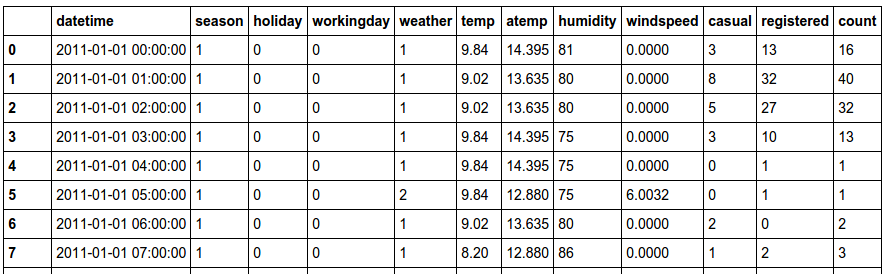### Engineer features for the model

Split the datetime field into date and time.

``````# engineer date and time features
temp = pd.DatetimeIndex(data['datetime'])
data['date'] = temp.date
data['time'] = temp.time
``````

Taking a closer look at the time feature, every reading is on the hour. So lets create an hour feature instead.

``````# create a feature called hour
data['hour'] = pd.to_datetime(data.time, format="%H:%M:%S")
data['hour'] = pd.Index(data['hour']).hour
``````

The date can tell us what day it is, so lets create a feature dayofweek. It also tells us how much time has passed since the survey began, which could be useful if there is a general growth in rental volumes over time. So lets create a feature dateDays.

``````# there appears to be a general increase in rentals over time, so days from start should be captured
data['dateDays'] = (data.date - data.date).astype('timedelta64[D]')

# create a categorical feature for day of the week (0=Monday to 6=Sunday)
data['dayofweek'] = pd.DatetimeIndex(data.date).dayofweek
``````

We can look more closely at which days of the week impact bike rental volumes. For casual users, volumes are much greater on Saturday & Sunday. For registered users, volumes are much less on Sunday. So let’s create binary features that ask if it is Saturday or Sunday.

``````byday = data.groupby('dayofweek')
byday['casual'].sum().reset_index()
``````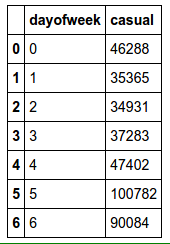``````byday['registered'].sum().reset_index()
``````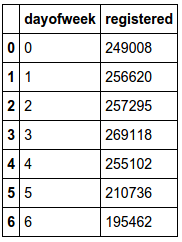``````# create binary features which show if day is Saturday/Sunday
data['Saturday']=0
data.Saturday[data.dayofweek==5]=1

data['Sunday']=0
data.Sunday[data.dayofweek==6]=1
``````

Finally, let’s remove redundant fields and see what we are left with.

``````# remove old data features
dataRel = data.drop(['datetime', 'count','date','time','dayofweek'], axis=1)
``````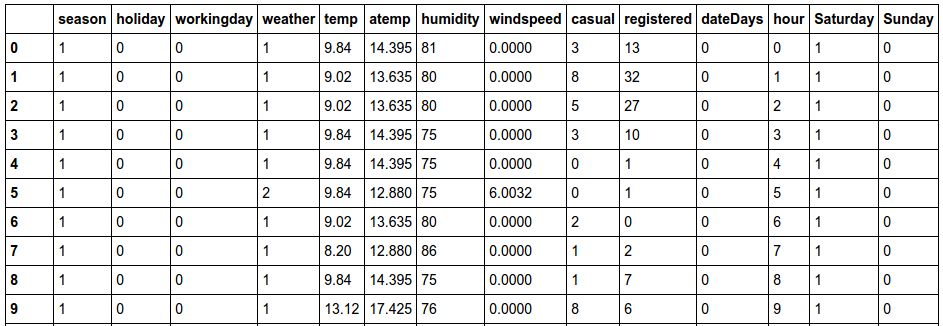### Vectorize features

All features must be in a vectorized format for the Scikit-learn models. We can achieve this by converting the Pandas dataframe to a dictionary, and then using a DictVectorizer from there.

We will split continuous and categorical features for now, so that we can prepare them differently in the next section.

``````# put continuous features into a dictionary
featureConCols = ['temp','atemp','humidity','windspeed','dateDays','hour']
dataFeatureCon = dataRel[featureConCols]
dataFeatureCon = dataFeatureCon.fillna( 'NA' ) #in case I missed any
X_dictCon = dataFeatureCon.T.to_dict().values()

# put categorical features into a dictionary
featureCatCols = ['season','holiday','workingday','weather','Saturday', 'Sunday']
dataFeatureCat = dataRel[featureCatCols]
dataFeatureCat = dataFeatureCat.fillna( 'NA' ) #in case I missed any
X_dictCat = dataFeatureCat.T.to_dict().values()

# vectorize features
vec = DictVectorizer(sparse = False)
X_vec_cat = vec.fit_transform(X_dictCat)
X_vec_con = vec.fit_transform(X_dictCon)
``````

### Standardize continuous features

Continuous features should be standardized to have zero mean and unit variance. This stops features with large numbers having too much impact on the models.

``````# standardize data - zero mean and unit variance
scaler = preprocessing.StandardScaler().fit(X_vec_con)
X_vec_con = scaler.transform(X_vec_con)
``````

### Encode categorical features

For example, before encoding the season field is one column containing either 1, 2, 3 or 4. After encoding, the season field will be represented by four binary fields for each option 1, 2, 3 or 4.

``````# encode categorical features
enc = preprocessing.OneHotEncoder()
enc.fit(X_vec_cat)
X_vec_cat = enc.transform(X_vec_cat).toarray()
``````

### Combine all features

After combining all features into one vector, we are ready to start training models!

``````# combine cat & con features
X_vec = np.concatenate((X_vec_con,X_vec_cat), axis=1)
``````

Below is a view of the first vectorized feature set. The first 6 are standardized continuous features, the rest are encoded categorical features.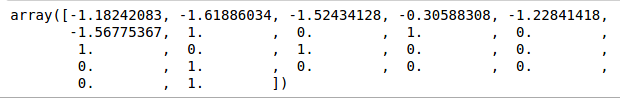### Vectorize targets

One final note, we must also create target vectors for the model.

``````# vectorize targets
Y_vec_reg = dataRel['registered'].values.astype(float)
Y_vec_cas = dataRel['casual'].values.astype(float)
``````

### Feedback

Always feel free to get in touch with other solutions, general thoughts or questions.